Next: The probabilistic model Up: Bayesian nonlinear state-space model Previous: Bayesian nonlinear state-space model   Contents

## The generative model

The Bayesian model follows the standard NSSM defined in Equation (4.13):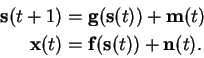(5.18)

The basic structure of the model can be seen in Figure 5.1.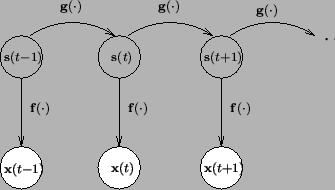The nonlinear functions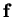and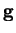of the NSSM are modelled by MLP networks. The networks have one hidden layer and can thus be written as in Equation (4.15):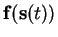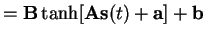(5.19)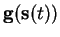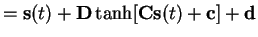(5.20)

where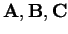and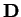are the weight matrices of the networks and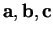and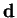are the bias vectors.

Because the data are assumed to be generated by a continuous dynamical system, it is reasonable to assume that the values of the hidden states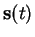do not change very much from one time index to the next one. Therefore the MLP network representing the functionis used to model only the change.Next: The probabilistic model Up: Bayesian nonlinear state-space model Previous: Bayesian nonlinear state-space model   Contents
Antti Honkela 2001-05-30